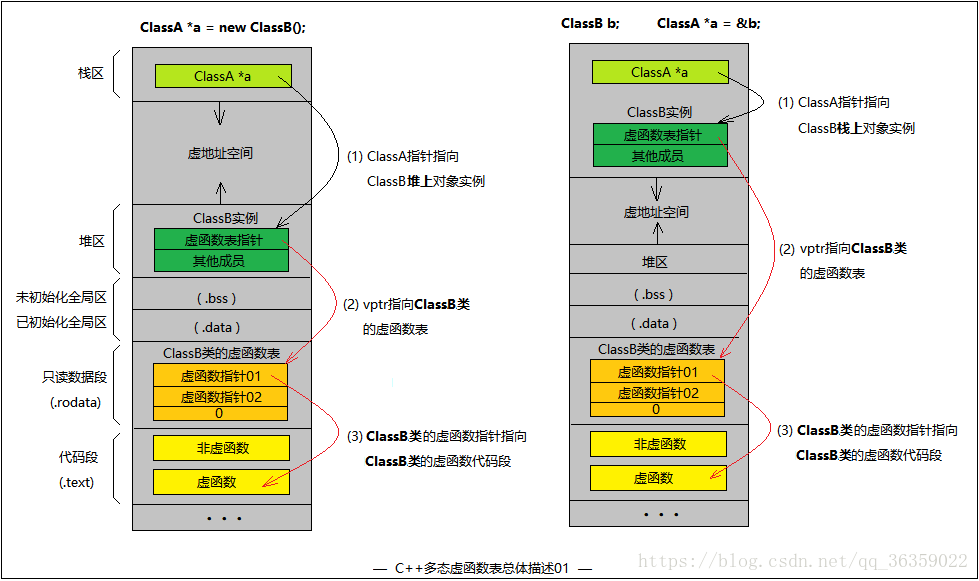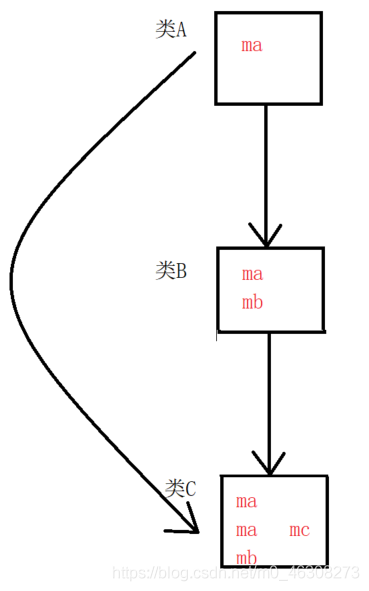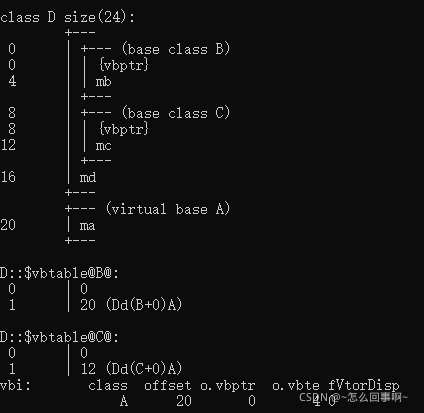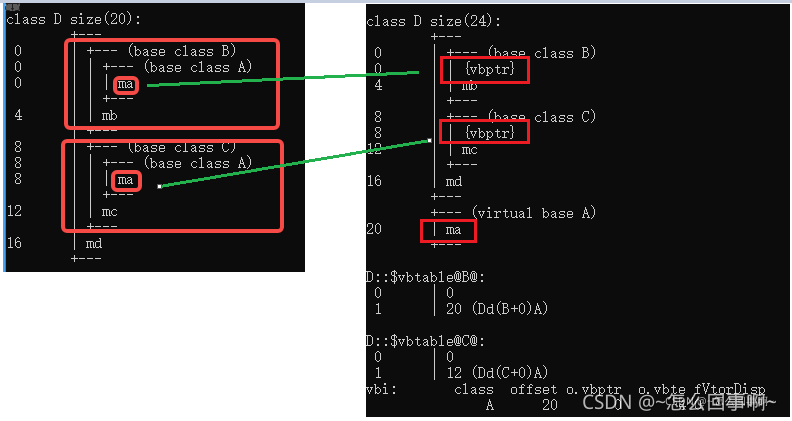# 關於C++虛函數與靜態、動態綁定的問題

## 不存在虛函數

```#include <iostream>
#include <typeinfo>
class Base
{
public:
Base(int data = 10): ma(data) {}
~Base() {};

void show() {
std::cout << "Base::show()" << std::endl;
}

void show(int data) {
std::cout << "Base::show()" << data << std::endl;
}

protected:
int ma;

};

class Derive :public Base
{
public:
Derive(int data) :Base(data), mb(data) {}
~Derive() {}
void show() {
std::cout << "Derive::show()" << std::endl;
}

private:
int mb;
};

int main() {

Derive d(50);
Base *pb = &d;
pb->show();//靜態（編譯時期）綁定(函數調用) Base::show (06F12E4h)
pb->show(10);//Base::show (06F12BCh)

std::cout << "Base size:" << sizeof(Base) << std::endl;//4
std::cout << "Derive size:" << sizeof(Derive) << std::endl;//8

std::cout << typeid(pb).name() << std::endl;//class Base *
std::cout << typeid(*pb).name() << std::endl;//class Base

return 0;

}```pb的類型是一個class Base *；
*pb的類型是一個class Base。Derive類繼承瞭Base類，導致瞭派生類的大小要比基類大，而pb的類型是基類的指針，所以通過pb調用方法時隻能訪問到Derive中從Base繼承而來的方法，訪問不到自己重寫的方法（指針的類型限制瞭指針解引用的能力）

## 基類定義虛函數

```#include <iostream>
#include <typeinfo>
class Base
{
public:
Base(int data = 10): ma(data) {}
~Base() {};

//虛函數
virtual void show() {
std::cout << "Base::show()" << std::endl;
}

void show(int data) {
std::cout << "Base::show()" << data << std::endl;
}

protected:
int ma;

};

class Derive :public Base
{
public:
Derive(int data) :Base(data), mb(data) {}
~Derive() {}
void show() {
std::cout << "Derive::show()" << std::endl;
}

private:
int mb;
};

int main() {

Derive d(50);
Base *pb = &d;

/*
pb->show();
pb 指針是base類型，如果發現Base中的show是虛函數，就進行動態綁定
mov         ecx,dword ptr [pb]
00292B01 8B 45 D4             mov         eax,dword ptr [pb]   //將pb指向的內存前4個字節放入ecx寄存器，pb指向derive對象，前四個字節即vfptr，將虛函數表地址加載到eax
00292B04 8B 10                mov         edx,dword ptr [eax]  //將eax 的前四個字節 即Derive::show 加載到edx中
00292B06 8B F4                mov         esi,esp
00292B08 8B 4D D4             mov         ecx,dword ptr [pb]
00292B0B 8B 02                mov         eax,dword ptr [edx]
00292B0D FF D0                call        eax   //虛函數的地址
00292B0F 3B F4                cmp         esi,esp
00292B11 E8 9C E7 FF FF       call        __RTC_CheckEsp (02912B2h)

eax寄存器裡存放的是什麼內容，編譯階段根本無從知曉，隻能在運行的時候確定；

pb->show(10);  如果發現show是普通函數，就進行靜態綁定 call Base::show

*/
pb->show();//
pb->show(10);//

std::cout << "Base size:" << sizeof(Base) << std::endl;//8
std::cout << "Derive size:" << sizeof(Derive) << std::endl;//12

std::cout << typeid(pb).name() << std::endl;//class Base *
/*
pb的類型：Base類型，查看Base中有沒有虛函數
（1）Base中沒有虛函數*pb識別的就是編譯時期的類型 *pb 就是Base類型
(2) Base中有虛函數，*pb識別的就是運行時期的類型 RTTI類型:Derive
*/
std::cout << typeid(*pb).name() << std::endl;//class Derive

return 0;

}```

• 首先，如果類裡面定義瞭虛函數，那麼編譯階段，編譯器給這個類類型產生一個唯一的vftable虛函數表，虛函數表中主要存儲的內容是：RTTI(Run-time Type Information)指針和虛函數的地址，當程序運行時，每一張虛函數表都會加載到內存的.rodata區；
• 一個類裡面定義瞭虛函數，那麼這個類定義的對象，在運行時，內存中會多存儲一個vfptr虛函數指針，指向瞭對應類型的虛函數表vftable；
• 一個類型定義的n個對象，他們的vfptr指向的都是同一張虛函數表；
• 一個類裡面虛函數的個數，不影響對象內存的大小(vfptr)，影響的是虛函數表的大小。
• 如果派生類中的方法和從基類繼承來的某個方法中返回值、函數名以及參數列表都相同，且基類的方法是virtual，那麼派生類的這個方法，自動處理成虛函數1、RTTI，存放的是類型信息，也就是（Base或者Derive）
2、偏移地址：虛函數指針相對於對象內存空間的偏移，一般vfptr都在0偏移位置
3、下面的函數時虛函數入口地址### VS的工具來查看虛函數表的有關信息

1 找到2 在打開的窗口中切換到當前工程所在目錄：

C:\Program Files (x86)\Microsoft Visual Studio\2017\Community>cd C:\Users\Admin\source\repos\C++test\

3 輸入命令：`cl XXX.cpp /d1reportSingleClassLayoutXX`(第一個XXX表示源文件的名字，第二個代表你想查看的類類型，我這裡就是`Derive`)(1) ClassA *a = new ClassB();
(2) ClassB b; ClassA *a = &b;2 類的虛函數表與類實例的虛函數指針3 多態代碼及多重繼承情況

```#include <iostream>

using namespace std;

class ClassA
{
public:
ClassA() { cout << "ClassA::ClassA()" << endl; }
virtual ~ClassA() { cout << "ClassA::~ClassA()" << endl; }

void func1() { cout << "ClassA::func1()" << endl; }
void func2() { cout << "ClassA::func2()" << endl; }

virtual void vfunc1() { cout << "ClassA::vfunc1()" << endl; }
virtual void vfunc2() { cout << "ClassA::vfunc2()" << endl; }
private:
};

class ClassB : public ClassA
{
public:
ClassB() { cout << "ClassB::ClassB()" << endl; }
virtual ~ClassB() { cout << "ClassB::~ClassB()" << endl; }

void func1() { cout << "ClassB::func1()" << endl; }
virtual void vfunc1() { cout << "ClassB::vfunc1()" << endl; }
private:
int bData;
};

class ClassC : public ClassB
{
public:
ClassC() { cout << "ClassC::ClassC()" << endl; }
virtual ~ClassC() { cout << "ClassC::~ClassC()" << endl; }

void func2() { cout << "ClassC::func2()" << endl; }
virtual void vfunc2() { cout << "ClassC::vfunc2()" << endl; }
private:
int cData;
};

int main()
{
ClassC c;

return 0;
}```

(1) ClassA是基類, 有普通函數: func1() func2() 。虛函數: vfunc1() vfunc2() ~ClassA()
(2) ClassB繼承ClassA, 有普通函數: func1()。虛函數: vfunc1() ~ClassB()
(3) ClassC繼承ClassB, 有普通函數: func2()。虛函數: vfunc2() ~ClassB()

ClassB繼承與ClassA，其虛函數表是在ClassA虛函數表的基礎上有所改動的，變化的僅僅是在子類中重寫的虛函數。如果子類沒有重寫任何父類虛函數，那麼子類的虛函數表和父類的虛函數表在內容上是一致的

```ClassA *a = new ClassB();
a->func1();                    // "ClassA::func1()"   隱藏瞭ClassB的func1()
a->func2();                    // "ClassA::func2()"
a->vfunc1();                   // "ClassB::vfunc1()"  重寫瞭ClassA的vfunc1()
a->vfunc2();                   // "ClassA::vfunc2()"```4、多繼承下的虛函數表 (同時繼承多個基類)

```#include <iostream>

using namespace std;

class ClassA1
{
public:
ClassA1() { cout << "ClassA1::ClassA1()" << endl; }
virtual ~ClassA1() { cout << "ClassA1::~ClassA1()" << endl; }

void func1() { cout << "ClassA1::func1()" << endl; }

virtual void vfunc1() { cout << "ClassA1::vfunc1()" << endl; }
virtual void vfunc2() { cout << "ClassA1::vfunc2()" << endl; }
private:
int a1Data;
};

class ClassA2
{
public:
ClassA2() { cout << "ClassA2::ClassA2()" << endl; }
virtual ~ClassA2() { cout << "ClassA2::~ClassA2()" << endl; }

void func1() { cout << "ClassA2::func1()" << endl; }

virtual void vfunc1() { cout << "ClassA2::vfunc1()" << endl; }
virtual void vfunc2() { cout << "ClassA2::vfunc2()" << endl; }
virtual void vfunc4() { cout << "ClassA2::vfunc4()" << endl; }
private:
int a2Data;
};

class ClassC : public ClassA1, public ClassA2
{
public:
ClassC() { cout << "ClassC::ClassC()" << endl; }
virtual ~ClassC() { cout << "ClassC::~ClassC()" << endl; }

void func1() { cout << "ClassC::func1()" << endl; }

virtual void vfunc1() { cout << "ClassC::vfunc1()" << endl; }
virtual void vfunc2() { cout << "ClassC::vfunc2()" << endl; }
virtual void vfunc3() { cout << "ClassC::vfunc3()" << endl; }
};

int main()
{
ClassC c;

return 0;
}```

ClassA1是第一個基類，擁有普通函數func1()，虛函數vfunc1() vfunc2()。
ClassA2是第二個基類，擁有普通函數func1()，虛函數vfunc1() vfunc2()，vfunc4()。
ClassC依次繼承ClassA1、ClassA2。普通函數func1(),虛函數vfunc1() vfunc2() vfunc3()。1.子類虛函數會覆蓋每一個父類的每一個同名虛函數。
2.父類中沒有的虛函數而子類有，填入第一個虛函數表中，且用父類指針是不能調用。
3.父類中有的虛函數而子類沒有，則不覆蓋。僅子類和該父類指針能調用

## 虛基類和多重繼承

### 什麼是多重繼承`類B``類C``類A`單繼承而來；
`類D``類B``類C`多繼承而來。

```#include <iostream>

using namespace std;
class A
{
public:
A(int data) :ma(data) { cout << "A()" << endl; }
~A() { cout << "~A()" << endl; }
protected:
int ma;
};
class B :public A
{
public:
B(int data) :A(data), mb(data) { cout << "B()" << endl; }
~B() { cout << "~B()" << endl; }
protected:
int mb;
};
class C :public A
{
public:
C(int data) :A(data), mc(data) { cout << "C()" << endl; }
~C() { cout << "~C()" << endl; }
protected:
int mc;
};
class D :public B, public C
{
public:
D(int data) : B(data), C(data), md(data) { cout << "D()" << endl; }
~D() { cout << "~D()" << endl; }
protected:
int md;
};
int main()
{
D d(10);

return 0;
}```（如果多繼承的數量增加，那麼派生類中重復的數據也會增加！）```class A
{
XXXXXX;
};
class B : virtual public A
{
XXXXXX;
};```

## 虛基類如何解決問題

```#include <iostream>

using namespace std;
class A
{
public:
A(int data) :ma(data) { cout << "A()" << endl; }
~A() { cout << "~A()" << endl; }
protected:
int ma;
};
class B :virtual public A
{
public:
B(int data) :A(data), mb(data) { cout << "B()" << endl; }
~B() { cout << "~B()" << endl; }
protected:
int mb;
};
class C :virtual public A
{
public:
C(int data) :A(data), mc(data) { cout << "C()" << endl; }
~C() { cout << "~C()" << endl; }
protected:
int mc;
};
class D :public B, public C
{
public:
D(int data) : B(data), C(data), md(data) { cout << "D()" << endl; }
~D() { cout << "~D()" << endl; }
protected:
int md;
};
````D(int data) :A(data), B(data), C(data), md(data) { cout << "D()" << endl; }``vfptr`（虛函數指針）指向瞭`vftable`（虛函數表）一樣，
`vbptr`（虛基類指針）指向瞭`vbtable`（虛基類表）。

vbtable（虛基類表）的佈局也如圖所示，